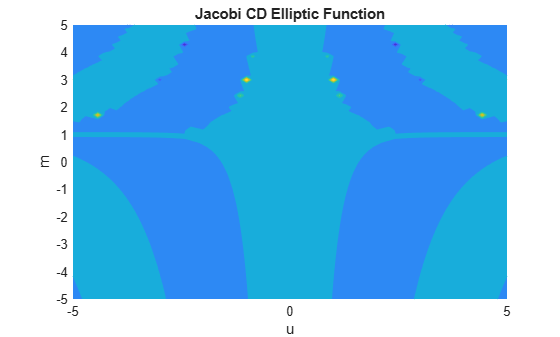# jacobiCD

Jacobi CD elliptic function

## Syntax

``jacobiCD(u,m)``

## Description

example

````jacobiCD(u,m)` returns the Jacobi CD Elliptic Function of `u` and `m`. If `u` or `m` is an array, then `jacobiCD` acts element-wise.```

## Examples

collapse all

`jacobiCD(2,1)`
```ans = 1```

Call `jacobiCD` on array inputs. `jacobiCD` acts element-wise when `u` or `m` is an array.

`jacobiCD([2 1 -3],[1 2 3])`
```ans = 1.0000 2.3829 -178.6290```

Convert numeric input to symbolic form using `sym`, and find the Jacobi CD elliptic function. For symbolic input where `u = 0` or `m = 0` or `1`, `jacobiCD` returns exact symbolic output.

`jacobiCD(sym(2),sym(1))`
```ans = 1```

Show that for other values of `u` or `m`, `jacobiCD` returns an unevaluated function call.

`jacobiCD(sym(2),sym(3))`
```ans = jacobiCD(2, 3)```

For symbolic variables or expressions, `jacobiCD` returns the unevaluated function call.

```syms x y f = jacobiCD(x,y)```
```f = jacobiCD(x, y)```

Substitute values for the variables by using `subs`, and convert values to double by using `double`.

`f = subs(f, [x y], [3 5])`
```f = jacobiCD(3, 5)```
`fVal = double(f)`
```fVal = 1.0019```

Calculate `f` to higher precision using `vpa`.

`fVal = vpa(f)`
```fVal = 1.0019475527333315357888731083364```

Plot the Jacobi CD elliptic function using `fcontour`. Set `u` on the x-axis and `m` on the y-axis by using the symbolic function `f` with the variable order `(u,m)`. Fill plot contours by setting `Fill` to `on`.

```syms f(u,m) f(u,m) = jacobiCD(u,m); fcontour(f,'Fill','on') title('Jacobi CD Elliptic Function') xlabel('u') ylabel('m')```## Input Arguments

collapse all

Input, specified as a number, vector, matrix, or multidimensional array, or a symbolic number, variable, vector, matrix, multidimensional array, function, or expression.

Input, specified as a number, vector, matrix, or multidimensional array, or a symbolic number, variable, vector, matrix, multidimensional array, function, or expression.

collapse all

### Jacobi CD Elliptic Function

The Jacobi CD elliptic function is

cd(u,m) = cn(u,m)/dn(u,m)

where cn and dn are the respective Jacobi elliptic functions.

The Jacobi elliptic functions are meromorphic and doubly periodic in their first argument with periods 4K(m) and 4iK'(m), where K is the complete elliptic integral of the first kind, implemented as `ellipticK`.

## Version History

Introduced in R2017b